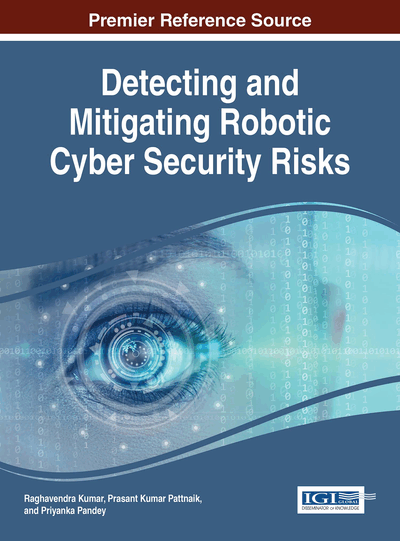# Digital Signature Schemes Based on Two Hard Problems

A. B. Nimbalkar (Annasaheb Magar Mahavidyalaya, India) and C. G. Desai (NDA, India)
DOI: 10.4018/978-1-5225-2154-9.ch007

## Abstract

This chapter takes a critical review of the digital signature schemes which are based on two hard problems. The analytical study begins with the Harn (1994) scheme and He-Kiesler (1994) scheme. Shao's 1998 and 2002 schemes have been studied. Wei-Hua He (2001) and Shimin Wei (2004, 2007) schemes are analyzed further in the research work. Attacks on Shimin Wei's (2004, 2007) schemes are critically studied and drawbacks have been noted so as to design better schemes than these. Then we continue our analysis work by studying Ismail, Thate, and Ahmad's (2008) scheme and Swati Verma's (2012) signature scheme.
Chapter Preview
Top

## Introduction

Scholars from cryptography and security field have done crucial of research work to design the robust and secure digital signature schemes till date. The key requirement of the digital signature is that it should sustain all the types of attacks it is exposed to. Designing the better digital signature scheme is one work whereas to make it tough and non-vulnerable to attacks is another biggest work. In this chapter, some of the digital signature schemes have been studied and analyzed thoroughly. This provides the guideline and a clear pathway, so that one can design a digital signature scheme, which is non-susceptible to known attacks.

The digital signature schemes that have been studied are the digital signature schemes designed in the period from the year 1994 till the year 2012, which were the golden years for security system design because internet and communication systems gained sudden big popularity in these two decades.

Here exiting digital signature schemes were studied and analyzed. In the analysis process, initially, Shimin Wei (2004, 2007) scheme and various known attacks on this scheme were taken up for the analysis. During the present research work process, in the year 2012 Swati Verma (2012) designed the digital signature scheme which came up with the solution for attack on Shimin Wei (2004) scheme. Then, the analysis focus was centered on Shimin Wei (2007) and Swati Verma (2012) schemes. Thorough analysis of digital signature algorithms namely Shimin Wei (2004, 2007) and Swati Verma (2012) was rigorously done along with their programming implementations. After the critical in depth analysis we showed an attack on Swati Verma (2012) scheme, this is one of the findings of our research work. The attack shown on Swati Verma (2012) scheme is message attack. Then the work was carried forward by proving this message attack by programming implementation and that forms the core part of novel approach to digital signature research work.

### L. Harn Scheme (1994)

L. Harn in 1994 developed a new efficient scheme based on two hard problems of factoring and discrete logarithms which enhanced the security of the scheme and at the same time could be effectively implemented in practice. The claim of Breaking the scheme required the solutions for two hard problems namely the Diffie-Hellman discrete logarithm problem in a subgroup of Zp* and factoring a certain integer into two large primes.

Digital signature scheme proposed by L. Harn can be divided into three phases: Initialization, Signature generation, and Signature verification.

#### Signature Verification

After receiving the message ‘‘ and signature (r, s), user can verify the signature. User first computes,

Next, user verifies the equation,

(2)

The performance of L. Harn scheme is near about the same as El Gamal scheme. The L. Harn signature requires one modular exponentiation for each message block and two modular exponentiations are required for deciphering each encrypted block. Since the encrypted blockis computed for each block of message, it increases the efficiency of transmission. Also security increases for every session since new encryption key is generated even if same key ‘‘ is used. The new encryption key is then shared by both the users.

One of the important aspects of L. Harn (1994) scheme is that it is effectively implemented in practice at the same time being more secure than El Gamal (1985) scheme.

## Complete Chapter List

Search this Book:
Reset